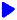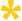Noetic Learning Math Worksheet Creator

Generate Word Problem WorksheetsBack to Worksheet Creator Home

Addition/Subtraction/Mixed - One Digit    free sampleOne Digit Addition Word Problems  Generate!One Digit Subtraction Word Problems  Generate!One Digit Mixed Word Problems  Generate!

Addition/Subtraction/Mixed - Two Digits    free sampleTwo Digits Addition Word Problems  Generate!Two Digits Subtraction Word Problems  Generate!Two Digits Mixed Word Problems  Generate!

Multiplication/Division/Mixed - One Digit    free sampleOne Digit Multiplication Word Problems  Generate!One Digit Division Word Problems  Generate!One Digit Mixed Word Problems  Generate!

Multiplication/Division/Mixed - Larger Numbers    free sampleLarger Number Multiplication Word Problems  Generate!Larger Number Division Word Problems   Generate!Large Number Mixed Word Problems  Generate!